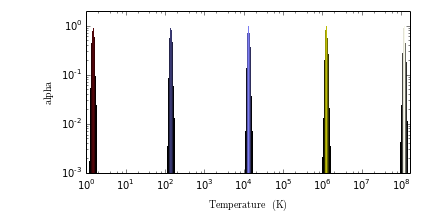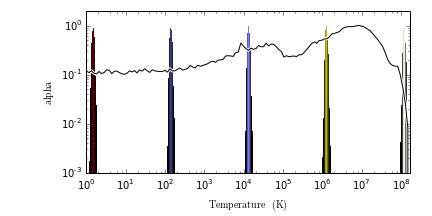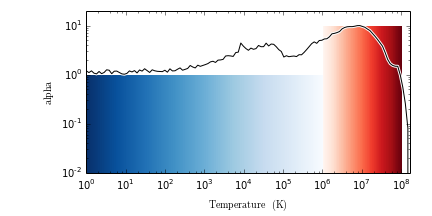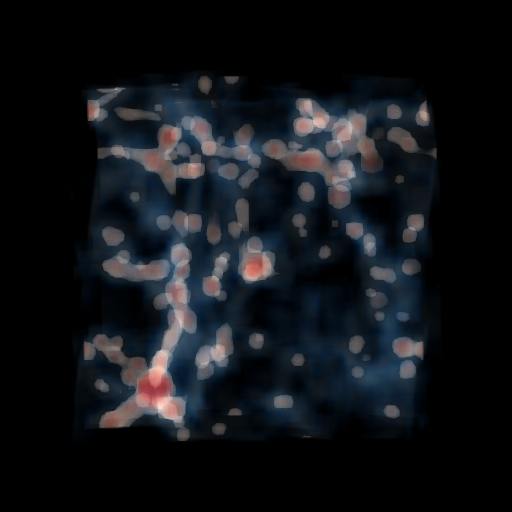# TransferFunctionHelper: Building Beautiful Transfer Functions¶

Here, we explain how to use TransferFunctionHelper to visualize and interpret yt volume rendering transfer functions. TransferFunctionHelper is a utility class that makes it easy to visualize he probability density functions of yt fields that you might want to volume render. This makes it easier to choose a nice transfer function that highlights interesting physical regimes.

First, we set up our namespace and define a convenience function to display volume renderings inline in the notebook. Using %matplotlib inline makes it so matplotlib plots display inline in the notebook.

In :
%matplotlib inline
from yt.mods import *
from IPython.core.display import Image
from yt.visualization.volume_rendering.transfer_function_helper import TransferFunctionHelper

def showme(np_im):
np_im[np_im != np_im] = 0.0
imb = write_bitmap(np_im, None)
return Image(imb)


Next, we load up a low resolution Enzo cosmological simulation.

In :
pf = load('Enzo_64/DD0043/data0043')


Now that we have the dataset loaded, let's create a TransferFunctionHelper to visualize the dataset and transfer function we'd like to use.

In :
tfh = TransferFunctionHelper(pf)


TransferFunctionHelpler will intelligently choose transfer function bounds based on the data values. Use the plot() method to take a look at the transfer function.

In :
# Build a transfer function that is a multivariate gaussian in Density
tfh = TransferFunctionHelper(pf)
tfh.set_field('Temperature')
tfh.set_log(True)
tfh.set_bounds()
tfh.build_transfer_function()
tfh.plot()

Out:Let's also look at the probability density function of the CellMass field as a function of Temperature. This might give us an idea where there is a lot of structure.

In :
tfh.plot(profile_field='CellMass')

Out:It looks like most of the gas is hot but there is still a lot of low-density cool gas. Let's construct a transfer function that highlights both the rarefied hot gas and the dense cool gas simultaneously.

In :
tfh = TransferFunctionHelper(pf)
tfh.set_field('Temperature')
tfh.set_bounds()
tfh.set_log(True)
tfh.build_transfer_function()
tfh.tf.add_layers(8, w=0.01, mi=4.0, ma=8.0, col_bounds=[4.,8.], alpha=np.logspace(-1,2,7), colormap='RdBu_r')
tfh.tf.map_to_colormap(6.0, 8.0, colormap='Reds', scale=10.0)
tfh.tf.map_to_colormap(-1.0, 6.0, colormap='Blues_r', scale=1.)

tfh.plot(profile_field='CellMass')

Out:Finally, let's take a look at the volume rendering.

In :
L = [-0.1, -1.0, -0.1]
c = pf.domain_center
W = 1.5*pf.domain_width
Npixels = 512
cam = pf.h.camera(c, L, W, Npixels, tfh.tf, fields=['Temperature'],
sub_samples=5, no_ghost=False, l_max=0)

# Here we substitute the TransferFunction we constructed earlier.
cam.transfer_function = tfh.tf

im = cam.snapshot()
showme(im[:,:,:3])

Out:## Now let's play with the "clip_ratio" kwarg in the snapshot function. This is supposed to modify the values of the returned image array to clip them at a N * the standard deviation of the array, which should allow you to "brighten" or "enhance" your resulting image.¶

In :
im2 = cam.snapshot(clip_ratio=2.0)
showme(im2[:,:,:3])

Out:In :
im3 = cam.snapshot(clip_ratio=20.0)
showme(im3[:,:,:3])

Out:In :
print (im == im2).all()
print (im == im3).all()

True
True